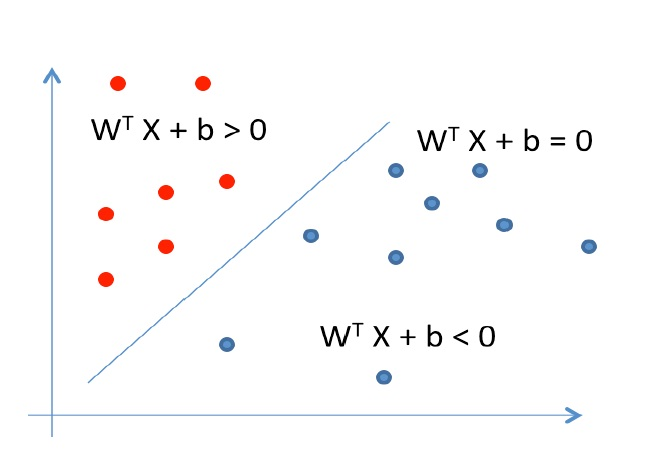## Support Vector Machine (SVM)

Support Vector Machine (SVM) is a very popular machine learning technique known for its accuracy, ease of application and versatility. This research does only apply SVM on the available feature set, so we try to explain only the basics about SVM. Vapnik and his team introduced SVM in 1992. Classification is one of the things that SVM is capable of doing. In classification the SVM is trained on a part of dataset known as training dataset which will have a certain discrete target value, and the input are continuous, and a small part of the data. SVMs find many applications in fields like pattern recognition, speech recognition, object recognition, time series prediction or forecasting, skin cancer detection, etc.Figure 3: SVMs representation

The above figure shows diagrammatic representation of working of linear SVM. The x coordinates for both red and blue dots represent one of the feature set and y co-ordinates represent the other feature, getting close to this research let us say that red dots represent ‘no profit’ and blue dots represent ‘profit’, and we are working on the training set, so the SVM classifier generates the hyper plane separating the two areas by using some complicated math. And finally seeing on which side of the hyper plane does each point fall can test the testing set.

Mathematically speaking, consider ? = ?!, ?! , ? = 1,2,3,….?
Where,
?! ∈ ?!
?! ∈ {−1, 1}

In simple words ? represents the data points or the training set and ? represents the known output labels, which for explanation purpose are taken as two, a actual problem may have more labels.
SVM satisfies the following conditions.figure 1

Task of the SVM classifier is to minimize | ? |! or maximize 1/| ? |. So a hyperplane is obtained with maximum margin from both sides.Table 1: SVM Kernels